NEET  >  Test: Kinematics of Rotational Motion

# Test: Kinematics of Rotational Motion

Test Description

## 20 Questions MCQ Test Physics Class 11 | Test: Kinematics of Rotational Motion

Test: Kinematics of Rotational Motion for NEET 2023 is part of Physics Class 11 preparation. The Test: Kinematics of Rotational Motion questions and answers have been prepared according to the NEET exam syllabus.The Test: Kinematics of Rotational Motion MCQs are made for NEET 2023 Exam. Find important definitions, questions, notes, meanings, examples, exercises, MCQs and online tests for Test: Kinematics of Rotational Motion below.
Solutions of Test: Kinematics of Rotational Motion questions in English are available as part of our Physics Class 11 for NEET & Test: Kinematics of Rotational Motion solutions in Hindi for Physics Class 11 course. Download more important topics, notes, lectures and mock test series for NEET Exam by signing up for free. Attempt Test: Kinematics of Rotational Motion | 20 questions in 20 minutes | Mock test for NEET preparation | Free important questions MCQ to study Physics Class 11 for NEET Exam | Download free PDF with solutions
 1 Crore+ students have signed up on EduRev. Have you?
Test: Kinematics of Rotational Motion - Question 1

### Direction of linear velocity of a particle rotating in a circular motion is :

Detailed Solution for Test: Kinematics of Rotational Motion - Question 1

Unlike linear motion, where velocity and acceleration are directed along the line of motion, in circular motion the direction of velocity is always tangent to the circle. This means that as the object moves in a circle, the direction of the velocity is always changing. A linear velocity of a particle performing circular motion, which is directed along the tangent to the circular path at given point on the circular path at that instant is called instantaneous velocity. It is also called as tangential velocity.

Test: Kinematics of Rotational Motion - Question 2

### A ball is under the effect of circular motion about a perpendicular axis with respect to the reference direction. The angular position (in radians) is given by the following function θ = t2 - 0.4t + 2 .The angular position when angular velocity is zero is

Test: Kinematics of Rotational Motion - Question 3

### Let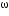H andE respectively be the angular speeds of the hour hand of a watch and that of the earth around its own axis. Compare the angular speeds of the earth and hour hand of a watch.

Detailed Solution for Test: Kinematics of Rotational Motion - Question 3

Given,
Angular velocity of the hour hand-H
Earth’s speed around its own axis-E
So, time taken by hour hand to complete a rotation is=TH=12hr
Time taken by earth to complete a rotation =TE=24 hours
So,
ωEH =(2π/TE )/2π/TH=TH/TE=1/2
⇒ ωEH=1/2
⇒ 2 ωE= ωH

Test: Kinematics of Rotational Motion - Question 4

The angular position of a particle (in radians), along a circle of radius 0.8 m is given by the function in time (seconds) by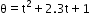. The linear velocity of the particle

Detailed Solution for Test: Kinematics of Rotational Motion - Question 4

So, as we know,
After differentiating angular velocity with respect to t,
Linear velocity=2t+2.3=ω
Now,
Velocity=r x ω(where r is 0.8m)
=0.8x2.3
=1.84m/s

Test: Kinematics of Rotational Motion - Question 5

All the points of a rigid body rotating about the given axis have same :

Detailed Solution for Test: Kinematics of Rotational Motion - Question 5

Thus, it is the velocity of a reference point fixed to the body. During purely translational motion (motion with no rotation), all points on a rigid body move with the same velocity. However, when motion involves rotation, the instantaneous velocity of any two points on the body will generally not be the same.

Test: Kinematics of Rotational Motion - Question 6

A mixer grinder rotates clockwise, its angular velocity will be :

Detailed Solution for Test: Kinematics of Rotational Motion - Question 6

Clock wise direction means inward field so angular velocity is negative. Anticlockwise means outward field so angular velocity is positive.

Test: Kinematics of Rotational Motion - Question 7

Which of the following is not a unit of angular displacement?

Detailed Solution for Test: Kinematics of Rotational Motion - Question 7

Angular displacement is measured in units of radians. 2 pi radians equals 360 degrees. The angular displacement is not a length (i.e. not measured in meters or feet), so an angular displacement is different than a linear displacement.

Test: Kinematics of Rotational Motion - Question 8

In an equilateral triangle of length 6 cm , three masses m1= 40g , m2= 60g and m3= 60g are located at the vertices. The moment of inertia of the system about an axis along the altitude of the triangle passing through mis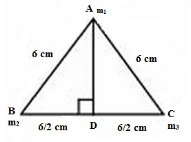Detailed Solution for Test: Kinematics of Rotational Motion - Question 8

Axis is along AD so Moment of inertia of first mass is zero and 2nd and 3rd masses are at distance of 6/2 from axis, so by using simply I=mr2 we get, I=60×36/4+60×36/4=1080 g cm2

Test: Kinematics of Rotational Motion - Question 9

A CD accelerates uniformly from rest to 200 spins per minute in 8 seconds. If the rate of change of speed is constant, determine the instantaneous acceleration in rad/s2 .

Detailed Solution for Test: Kinematics of Rotational Motion - Question 9

The correct option is A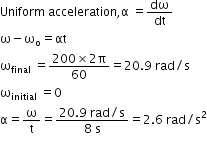Test: Kinematics of Rotational Motion - Question 10

A boy is playing with a tire of radius 0.5m. He accelerates it from 5rpm to 25 rpm in 15 seconds. The linear acceleration of tire is

Detailed Solution for Test: Kinematics of Rotational Motion - Question 10

r=0.5m, t=15s
n1=5rpm=5/60 rps
n2=25rpm=25/60rps
As,
a=r∝          [∝=ω2- ω1/t]
so, a=0.5[2π(n2-n1)/t]
a=0.5x6.28x20/60x15
a=6.28x2/60x3
a=6.28/90
a=0.697 m/s2
a≈0.7 m/s2

Test: Kinematics of Rotational Motion - Question 11

The angular momentum of a body is 31.4 Js and its rate of revolution is 10 cycle per second. The momentum of inertia of the body about the axis of rotation is

Detailed Solution for Test: Kinematics of Rotational Motion - Question 11

F = w/2π
w = 2πf
w = 2π × 10
w = 62.8
L = Iw
I = L/w
I = 31.4/62.8
I = 0.5 kgm2
Hence A

Test: Kinematics of Rotational Motion - Question 12

A merry- go- round ( diameter 4.0 m ) starts from rest and uniformly accelerates until it rotates at 0.8 rev/min. This occurs in 11s. Once it has reached this rate , it rotates at 0.8 rev/min uniformly . Determine the angular acceleration

Detailed Solution for Test: Kinematics of Rotational Motion - Question 12

First we have to find ‘v’ by using the relation v = rw
Now, v = 0.167m/s
Then we find ‘a’ by using the relation a = v/t
So, a = 0.0152m/s2.
Now, we can find angular acceleration by using the formula α = a/r

Test: Kinematics of Rotational Motion - Question 13

A demo CD, 8 cm in diameter, spins 200 rev/min. Determine its linear velocity in cm/s for a point 3 cm from the center.

Test: Kinematics of Rotational Motion - Question 14

The Equations of Rotational Motion are

Detailed Solution for Test: Kinematics of Rotational Motion - Question 14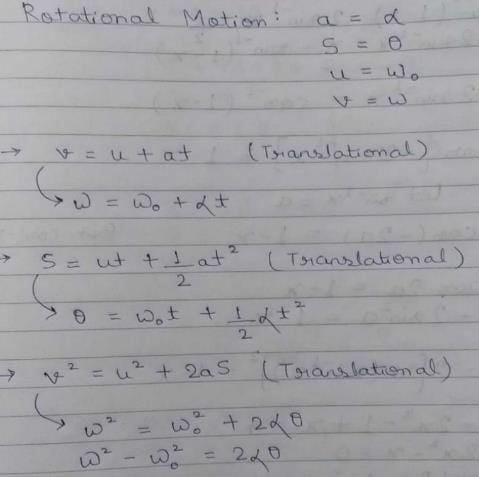Test: Kinematics of Rotational Motion - Question 15

Rotational analogue of force is :

Detailed Solution for Test: Kinematics of Rotational Motion - Question 15

The equation τ = mr2α is the rotational analog of Newton's second law (F=ma), where torque is analogous to force, angular acceleration is analogous to translational acceleration, and mr2 is analogous to mass (or inertia).

Test: Kinematics of Rotational Motion - Question 16

A wheel rotates with a constant acceleration of 2.0 rad/s2 . If the wheel starts from rest, the number of revolutions it makes in the first ten seconds will be approximately:

Detailed Solution for Test: Kinematics of Rotational Motion - Question 16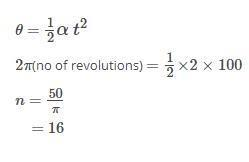Test: Kinematics of Rotational Motion - Question 17

The angular velocity of a second hand of a clock is :

Detailed Solution for Test: Kinematics of Rotational Motion - Question 17

Time taken to complete one rotation is 60 seconds.
One rotation involves 360 degree = 2π
Formula of angular velocity = theta/time taken
Hence, omega = 2π/60

Test: Kinematics of Rotational Motion - Question 18

A car is decelerating uniformly from velocity v1 to velocity v2 while getting displaced by s. If the diameter of the wheel is d, the angular acceleration of the wheel is

Test: Kinematics of Rotational Motion - Question 19

A smooth sphere A is moving on a frictionless horizontal plane with angular speed ω and center of mass velocity v. It collides elastically and head on with an identical sphere B at rest. Neglect friction everywhere. After the collision, their angular speeds are ωA and ωB respectively. Then

Detailed Solution for Test: Kinematics of Rotational Motion - Question 19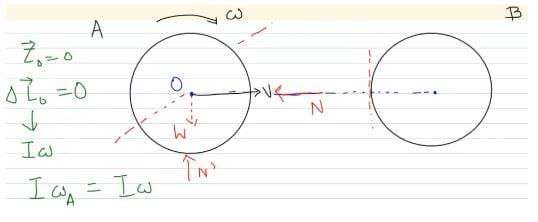So, “I” gets cancelled.

Test: Kinematics of Rotational Motion - Question 20

If n is the frequency of rotation of a body, its angular velocity is:

## Physics Class 11

127 videos|464 docs|210 tests
 Use Code STAYHOME200 and get INR 200 additional OFF Use Coupon Code
Information about Test: Kinematics of Rotational Motion Page
In this test you can find the Exam questions for Test: Kinematics of Rotational Motion solved & explained in the simplest way possible. Besides giving Questions and answers for Test: Kinematics of Rotational Motion, EduRev gives you an ample number of Online tests for practice

## Physics Class 11

127 videos|464 docs|210 tests

### How to Prepare for NEET

Read our guide to prepare for NEET which is created by Toppers & the best Teachers